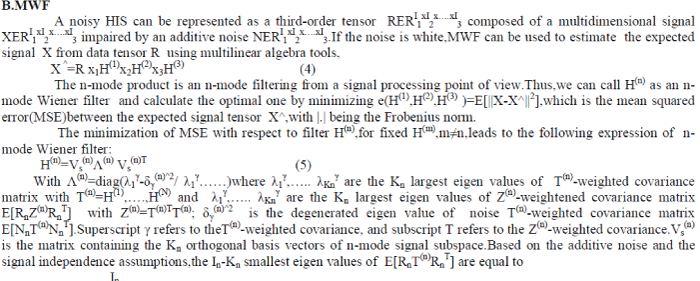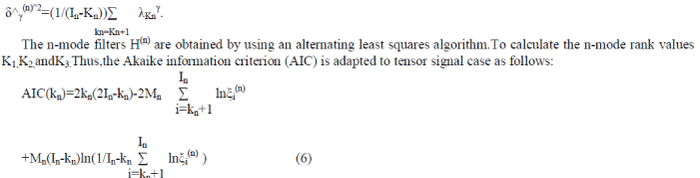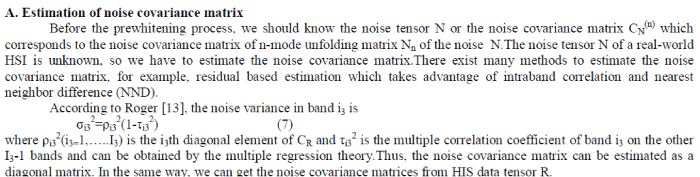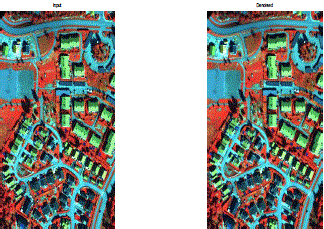ISSN ONLINE(2320-9801) PRINT (2320-9798)

All submissions of the EM system will be redirected to Online Manuscript Submission System. Authors are requested to submit articles directly to Online Manuscript Submission System of respective journal.

# Nonwhite Noise Reduction In Hyperspectral Images

 Mrs.Suryatheja.M.B and Gokilamani.R P.G.Scholars, I Year, Department of ECE, SNS College of Engineering, Coimbatore, Tamilnadu, India Related article at Pubmed, Scholar Google

Visit for more related articles at International Journal of Innovative Research in Computer and Communication Engineering

## Abstract

Noise reduction is an important preprocessing step to analyze the information in hyperspectral image (HSI).Because the common filtering methods for HSIs is based on the data vectorization or matricization while ignoring the related information between image planes, there are new approaches considering the multidimensional data as whole entities.For example, Multidimensional Wiener filtering (MWF) based on the third order tensor decomposition. To reduce the nonwhite noise from HSIs, the first step is to whiten the noise in HSIs through a prewhitening procedure. Then MWF can help to denoise the prewhitened data.Atlast an inverse prewhitening process can rebuild the estimated signal.

### Keywords

Hyper spectral images, Multiway Filtering, Noise Reduction, and Nonwhite Noise.

### INTRODUCTION

Hyperspectral image normally consists of hundreds of spectral bands and is also named as a tensor.Acquired Hyper spectral images are disturbed by additive noise,which impairs the useful information and disturbs the scene interpretation.Denoising is of target classification or detection with the underlying principle of targets being distinguishable.The noise mainly comes from two aspects:signal dependent (SD)and signal independent(SI) photonic noise.Although the SD photonic noise has become as dominant as SI circuitry noise,the additive SI noise is still an important part of noise.Since the denoising methods for those two types of noise are not the same.we mainly focus on the reduction of additive SI noise. Hyperspectral imaging, like other spectral imaging, collects and processes information from across the electromagnetic spectrum. Much as the human eye sees visible light in three bands (red, green, and blue), spectral imaging divides the spectrum into many more bands. This technique of dividing images into bands can be extended beyond the visible.
The tensor decomposition method has been used to denoise those images and showed some prospects in this field.Two main decomposition models for multi dimensional arrays have been developed:Tucker3 decomposition and canonical decomposition/parallel factor analysis decomposition.We focus on tucker3 decomposition, is used to calculate higher order singular value decomposition and lower rank tensor approximation.It can distinguish between signal subspace and noise subspace by taking a Multidimensional data set as whole entity and considering the relationship between modes.MWF is more efficient than classical channel by channel wiener filtering or other Multidimensional filtering methods to denoise data sets with additive white noise.However,this filtering methods does not consider the cases with nonwhite noise.Thus we propose a prewhitening approach for HSIs,which could change the nonwhite noise to white one, then MWF can be used to filter the prewhitened HSIs .

#### MULTILINEAR ALGEBRA

Multilinear algebra extends the methods of linear algebra. Just as linear algebra is built on the concept of a vector and develops the theory of vector spaces, multilinear algebra builds on the concepts of p-vectors and multivectors with Grassmann algebra. The topic of multilinear algebra is applied in some studies of multivariate calculus and manifolds where the Jacobian matrix comes into play. The infinitesimal differentials of single variable calculus become differential forms in multivariate calculus, and their manipulation is done with exterior algebra.

### MULTILINEAR ALGEBRA TOOLS### MULTIDIMENSIONAL FILTERING

#### A.Data model

The model of a multidimensional tensor X disturbed by an additive noise tensor N is defined as
R=X+N     (3)
Denoising is to reduce the noise in the noisy R and estimate the expected signal through a multidimensional filtering of R.we assume that the noise N is independent from the signal X and the n mode rank Kn is smaller than the n-mode dimension In(Kn<In,for all n=1 to N,that is to save,the signal subspace has a rank smaller than the data space).we also suppose that,what ever the n-mode,the signal subspace is orthogonal to the noise subspais necessaryce.the signal subspace is spanned by the Kn singular vectors associated with the Kn largest singular values of matrix Rn.The noise subspace is spanned by the In-Kn singular vectors associated with theIn-Kn smallest singular values of Rn.where ξi (n) ,i=1,to In are the In eigen values of the covariance matrix E[RnRn T]of the n-mode unfolding matrix Rn of tensor R ,with ξi (n) ≥…≥ ξIn (n) .Mn is the number of columns of Rn as defined in (1).The estimated n-mode rank Kn is the value of kn which minimizes the Akaike information criteria(AIC).
However, there is an immediate drawback of this algorithmn: It deals only with white noise.Thus, in the case of general noise, particularly the nonwhite noise, a prewhitening process is necessary  to efficiently denoise the HIS using this MWF method. In the next case. We propose an extension of MWF which could deal with the HSIs disturbed by nonwhite noise.

### SOLUTION FOR NONWHITE NOISE

If the noise in HIS is not white, MWF cannot effectively remove the nonwhite noise and estimate the expected signal. In this case, we propose to change the nonwhite noise in R into a white one by a preprocessing procedure, then MWF can be used to denoise the whitened data tensor R..#### C.Denoising Algorithmn PMWF

The complete algorithmn for the reduction of nonwhite noise in HSIs can be formulated based on prewhitening and MWF as follows:
1) Unfolding tensor R to matrix Rn=Xn+Nn, n=1…N, estimating the noise covariance matrix CN (n) from R.
2) QR decomposition of Nn:Nn=QnPn;
3) Prewhitening Rn: Ȓn=RnPn -1.
4) Initialisation k=0.
5) While a) Estimation of n-mode ranks Kn,
n=1…N, KN=arg min [AIC(kn)].
b) Estimation of n-mode filters H(n)
6) Inverse procedure of prewhitening.

### EXISTING SYSTEM

Generalized multidimensional Wiener filter for denoising is adapted to hyperspectral images (HSIs). Commonly, multidimensional data filtering is based on data vectorization or matricization.

### PROPOSED SYSTEM

Multidimensional Wiener filtering (MWF) is one of the techniques that consider a multidimensional data set as a third-order tensor. It also relies on the separability between a signal subspace and a noise subspace. Using multilinear algebra, MWF needs to flatten the tensor.

#### Signal subspace & Noise subspace

In signal processing, signal subspace methods are empirical linear methods for dimensionality reduction and noise reduction. Essentially the methods represent the application of a principal components analysis (PCA) approach to ensembles of observed time-series obtained by sampling, for example sampling a video signal. Such samples can be viewed as vectors in a high-dimensional vector space over the real numbers. PCA is used to identify a set of orthogonal basis vectors (basis signals) which capture as much as possible of the energy in the ensemble of observed samples. The vector space spanned by the basis vectors identified by the analysis is then the signal subspace. The underlying assumption is that information in image signals is almost completely contained in a small linear subspace of the overall space of possible sample vectors, whereas additive noise is typically distributed through the larger space isotropically (for example when it is white noise

### EXPERIMENTS

#### Classification of Real-world HSI

The PMWF is tested on real world HIS which is distributed by noise because of some properties of imaging system. To know the noise characteristics of this HYDICE HIS, its noise covariance matrix is estimated according to this section IV.Fig.2 shows the noise reduction outcome which corresponds to the diagonal element of noise covariance matrix CN .It is clear that the noise in the HYDICE HIS is not white, so the prewhitening process is necessary.Fig. Imaging system noise reduction outcome.

### CONCLUSION

To reduce the nonwhite noise in HSI, a novel method, PMWF, is proposed by a two-stage process composed of a noise-prewhitening procedure and an MWF process. Its ability as a preprocessing procedure that improves SNRout, and SAM classification results applied to real-world HYDICE data.Quantitative results based on OA criterion show the effectiveness of this method. The comparison to MWF, PCA, PCA-Wiener, MNF, and MNF-Wiener permits to appreciate the denoising efficiency of our method in the application of target classification in noisy HYDICE HSI.